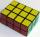Regular prism

The regular four-sided prism has a base of 25 cm2 and a surface of 210 cm2. Find its volume.

Result

V =  200 cm2

Solution:Leave us a comment of example and its solution (i.e. if it is still somewhat unclear...):Be the first to comment!To solve this example are needed these knowledge from mathematics:

Tip: Our volume units converter will help you with converion of volume units.

Next similar examples:

1. Prism 4 sidesFind the surface area and volume four-sided prism high 10cm if its base is a rectangle measuring 8 cm and 1.2dm
2. Triangular prismCalculate the surface area and volume of a triangular prism, base right triangle if a = 3 cm, b = 4 cm, c = 5 cm and height of prism h=12 cm.
3. Triangular prismCalculate the volume of a triangular prism 10 cm high, the base of which is an equilateral triangle with dimensions a = 5 cm and height va = 4,3 cm
4. Square prismA square prism has a base with a length of 23 centimeters, what is the area in square centimeters of the base of the prism?
5. FlowerbedThe flowerbed has a length 3500mm and a width 1400mm. How many foil is needed to covers the flowerbed? How many m2 of foil was consumed for its production (add 10% of the material to the joint and waste)? How many liters of air is inside the enclosure? (F
6. Rectangular prismIf i have a rectangular prism with a length of 1,000 cm, width of 30 cm and a height of 50 cm, what is the volume?
7. Rotary cylinder 2Base circumference of the rotary cylinder has same length as its height. What is the surface area of cylinder if its volume is 250 dm3?
8. GreenhouseGarden plastic greenhouse is shaped half cylinder with a diameter of 6 m and base length 20 m. At least how many m2 of plastic is need to its cover?
9. Cube wallsFind the volume and the surface area of the cube if the area of one of its walls is 40 cm2.
10. CubeThe sum of all cube edges is 30cm. Find the surface area of the cube.
11. Cube 1-2-3Calculate the volume and surface area of the cube ABCDEFGH if: a) /AB/ = 4 cm b) perimeter of wall ABCD is 22 cm c) the sum of the lengths of all edges of the cube is 30 cm.
12. Cuboid surfaceDetermine surface area of cuboid if its volume is 52.8 cm cubic and length of the two edges are 2 cm and 6 cm.
13. Cuboid - simpleCalculate the surface area and volume of a cuboid if a = 8 cm, b = 14 cm and c = 6 cm.
14. Cylinder twiceIf the radius of the cylinder increases twice, and the height is reduced twice, then the volume of the cylinder increases (how many times?):
15. Greg and BillGreg is 18 years old. He is 6 less than 4 times Bill's age. How old is Bill?
16. Simplify 2Simplify expression: 5ab-7+3ba-9
17. Simple equationsSolve system of equations: 5x+3y=5 5x+7y=25